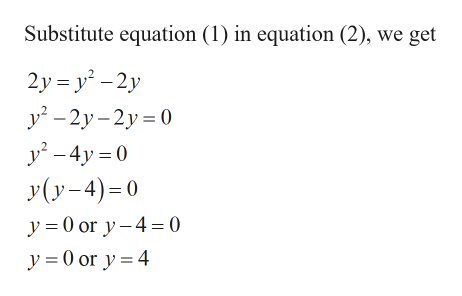Question
5 views

Give the solution to the system of equations.

x=2y x=y2-2y

check_circle

Step 1

Given:

Step 2

Calculation:

...help_outlineImage TranscriptioncloseSubstitute equation (1) in equation (2), we get 2y = y² - 2y y – 2y– 2y = 0 y – 4y = 0 y(y-4)=0 y = 0 or y- 4= 0 y = 0 or y = 4 fullscreen

### Want to see the full answer?

See Solution

#### Want to see this answer and more?

Solutions are written by subject experts who are available 24/7. Questions are typically answered within 1 hour.*

See Solution
*Response times may vary by subject and question.
Tagged in

### Equations and In-equations